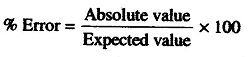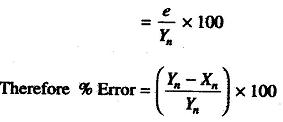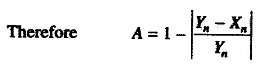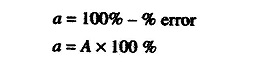## Errors in Measurement:

Errors in Measurement is the process of comparing an unknown quantity with an accepted standard quantity. It involves connecting a measuring instrument into the system under consideration and observing the resulting response on the instrument.

The measurement thus obtained is a quantitative measure of the so called “true value” (since it is very difficult to define the true value, the term “expected value” is used). Any measurement is affected by many variables, therefore the results rarely reflect the expected value. For example, connecting a measuring instrument into the circuit under consideration always disturbs (changes) the circuit, causing the measurement to differ from the expected value.

Some factors that affect the measurements are related to the measuring instruments themselves. Other factors are related to the person using the instrument. The degree to which a measurement nears the expected value is expressed in terms of the Errors in Measurement.

Error may be expressed either as absolute or as percentage of error. Absolute error may be defined as the difference between the expected value of the variable and the measured value of the variable, or

e = Yn – Xn

where

• e = absolute error
• Yn = expected value
• Xn = expected value

ThereforeIt is more frequently expressed as a accuracy rather than error.where A is the relative accuracy.

Accuracy is expressed as % accuracywhere a is the % accuracy.

Scroll to Top